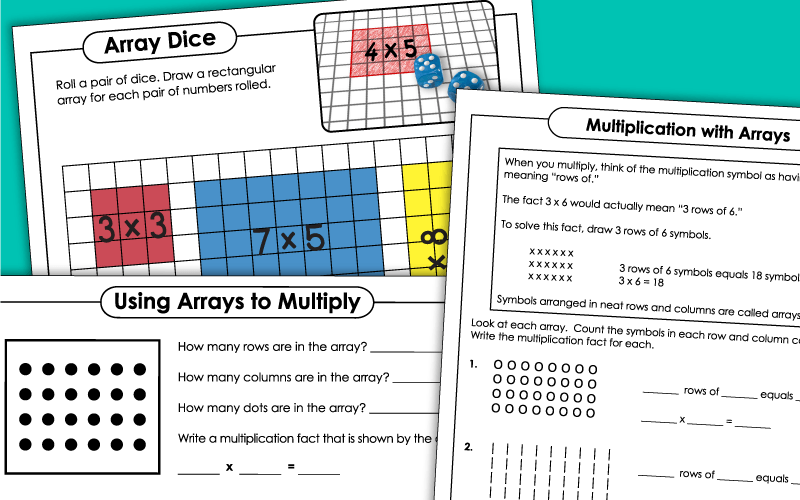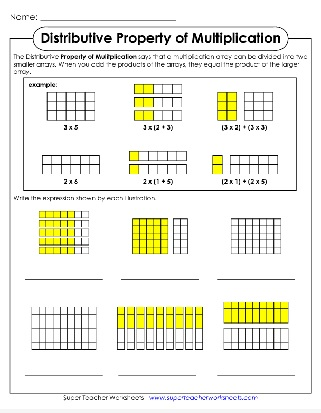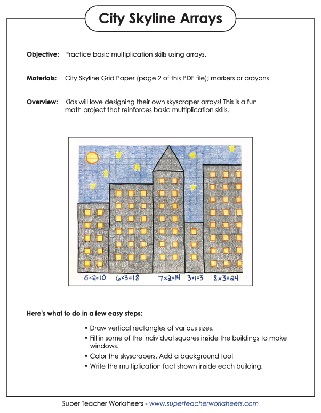# Multiplication & Division with Arrays

Learn about using arrays for multiplication and division with these printables worksheets, task cards, and activities.## Multiplication with Arrays:Worksheets

Examine each array illustration. Tell how many rows, how many columns, and how many dots. Then write a multiplication fact for each.
On this worksheet, your students will practice using arrays to solve multiplication facts. Count the row and columns to solve.
Here's a table with 100 stars. Numbers 1 through 10 are listed on the x and y axis. Use the L-shaped tool to make an array for any basic multiplication fact.
Cut out the multiplication facts, products, and array illustrations. Glue each in the correct position on the table.
Teach students to draw their own arrays. They are given a multiplication fact with space to draw circles in neat rows and columns.
Kids will love making these creative city skylines using graph paper! This activity helps them practice basic multiplication skills using arrays.
Draw arrays for any multiplication facts on this template graphic organizer.

## Distributive Property ofMultiplication Using Arrays

This worksheet uses arrays to make it easy for students to understand the distributive property of multiplication.
Here's another worksheet that uses arrays to help students understand the distributive property of multiplication.

## Multiplication with Arrays:Math Games & Task Cards

Cut out the 36 cards in this set. Match the facts, array graphics, and answers/products. You can also use this set to play a memory-match game.
Roll a pair of dice. Draw a rectangular array for each pair of numbers rolled.
Each of these 30 task cards has a question related to multiplication with arrays. Use them for classroom scavenger hunts, learning centers, or exit slips. They work great with document cameras too.

## Division with Arrays

Answer the questions for each array shown. Then find and solve the division fact.
Write the basic division fact shown by each array.

## Fact Families(Multiplication & Division)

Students are shown an array. They write the four multiplication and division facts for each.
Basic Multiplication Worksheets

On this page, you'll find a link to hundreds of worksheets and printable games for teaching basic multiplication facts.

## Sample Worksheet ImagesMy Account
Site Information